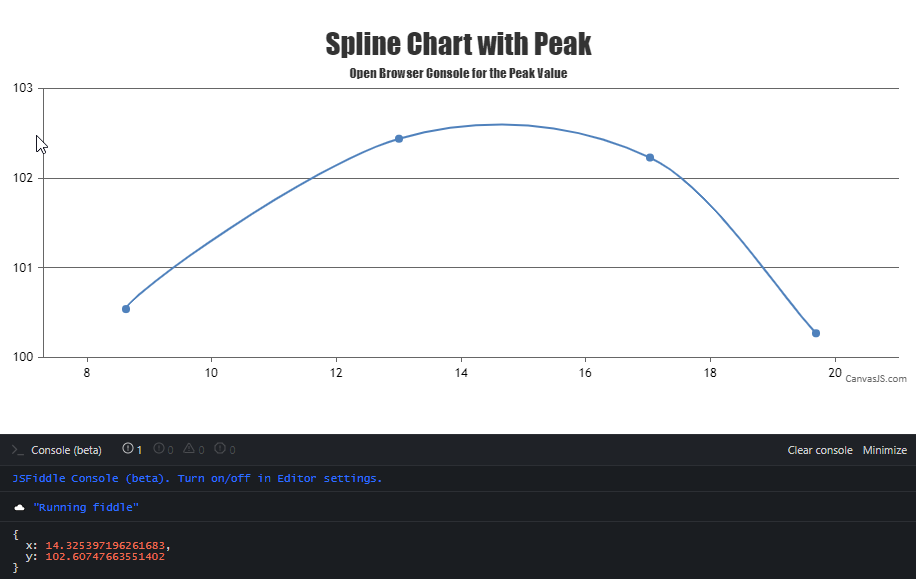Home Forums Chart Support Find max peak on spline chart Reply To: Find max peak on spline chart

#34501

CanvasJS uses Bézier curve interpolation to represent the curve passing through the given dataPoints. We don’t have any property to find the coordinates of the highest / lowest peak as of now. However, you can achieve the same by getting the image from the rendered chart & processing it to find the colored pixel-coordinate from top to bottom. Later you can use convertPixelToValue to get the value corresponding to the pixel-coordinate as shown in the code-snippet below,

``````var imageData = chart1.ctx.getImageData(chart1.plotArea.x1, chart1.plotArea.y1, chart1.plotArea.width, chart1.plotArea.height).data;

coloredRegionData = [];
for (var i = 0; i < imageData.length; i += 4) {
if (i % (4 * chart1.plotArea.width) === 0) {
coloredRegionData.push([]);
temp = i;
}
if (imageData[i] === 255 && imageData[i+1] === 0) {
coloredRegionData[coloredRegionData.length - 1].push((i - temp) / 4);
}
}

splinePixelCordinates = [];
for (var i = 0; i < coloredRegionData.length; i++) {
for (j = 0; j < coloredRegionData[i].length; j++) {
splinePixelCordinates.push({
x: chart1.plotArea.x1 + coloredRegionData[i][j],
y: chart1.plotArea.y1 + i
})
}
} ``````

Please take a look at this JSFiddle for an example on finding the coordinates of the highest / lowest peak.